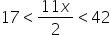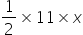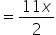Mathematics
Easy

Question

# Find the area of the right-angled triangle if the height is 11 units and the base is x units, given that the area of the triangle lies between 17 and 42 sq. units

##A > 17A ≤ 4217 ≤ 11x ≤ 42Hint:

## The correct answer is:### The height of the right-angled triangle is 11 units.The base of the right-angled triangle is x units.The area lies between the 17sq. units and 42sq. units. So, this question is of inequality. We have to write the mathematical form of this inequality.The area of a right-angled triangle is given as follows:Area =Now, we will see the limits.The area lies between 17sq. units and 42sq. units.It means the area should be greater than 17sq. units and less than 42sq. units.So, we can write17 < Area < 42Tbis is the given inequality.

For such questions, we should check the limits of the inequality. We should check the upper limit and lower limit.

### Related Questions to study#### With Turito Foundation.#### Get an Expert Advice From Turito.